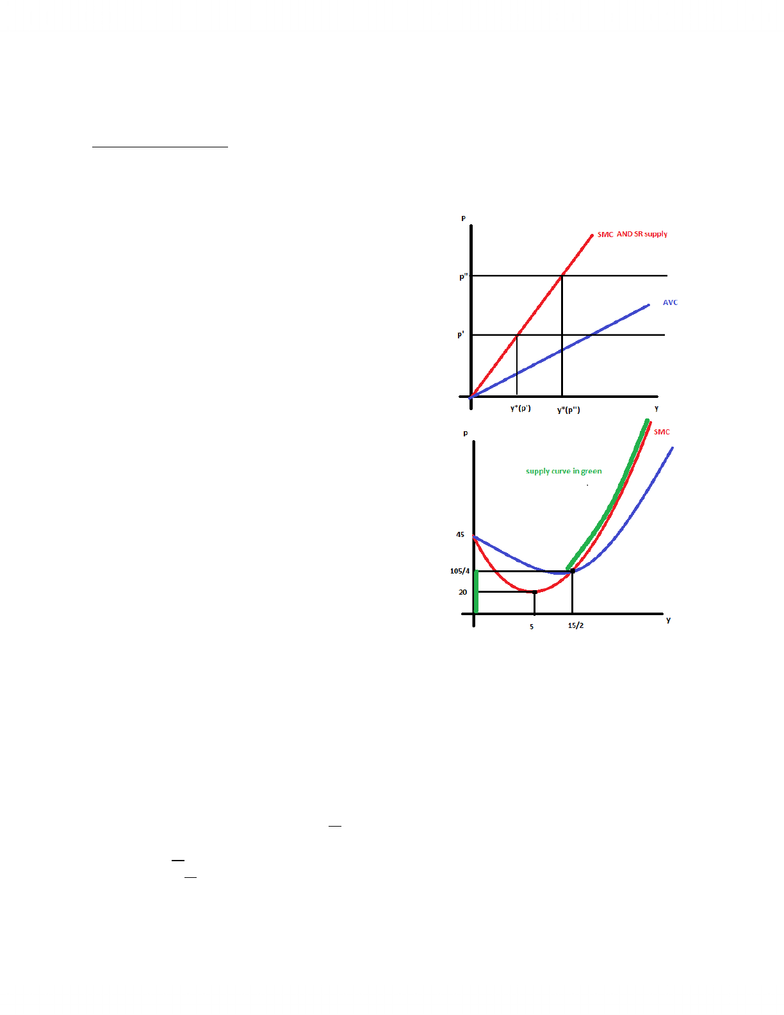# ECON 2X03 Lecture Notes - Lecture 4: Production Function, Marginal Product, Farad

27 views3 pages
School
McMaster University
Department
Economics
Course
ECON 2X03
ProfessorLecture 4
Individual Firm’s Supply
The supply function for a firm gives the profit maximizing quantity of output as a function of market
price. Found where SMC = P and SMC is upward-sloping and P>min of AVC (if P<min AVC, y = 0)
Ex. A firm has y = z11/2z21/2 and faces w1=w2=1. In the short run z2=4. What is its SR supply function?
- y=z11/241/2
- z1*= y2/4
- VC = w1z1* = y2/4
- SMC = dVC/dy = y/2
- SMC always upward-sloping
- AVC = VC/y=y/4 which is <y/2 = SMC for Vy>0
- So just set SMC = P
- y/2=P
- y*=2P
Ex. A firm has VC = y3/3-5y2+45y. What is the SR supply?
- SMC = dVC/dy = y2-10y+45
- dSMC/dy = 2y-10
- y= 5
- At y=5, SMC = 25-50+45 = 20
- AVC = VC/y = y2/3-5y+45
- dAVC/dy=2y/3-5 = 0
- y=15/2
- AVC(15/2) = 75/4-150/4+180/4=105/4
- So for P<=105/4, y*=0. For P>=105/4, P=SMC
- P = y2-10y+45
- y2-10y+45-P=0
- y2-10y+25+20-P=0
- (y-5)2+20-P=0
- (y-5)2=P-20
- Y=5+sqrt(P-20)
- So supply function: y* = {0 if P<=105/4 || 5+sqrt(P-20) if P>=105/4}
Market Supply Function (SR): total desired (π-maxing) output in a market as a function of market price.
Given a market with n firms with individual supply functions yi*(p) i=1,2,…n, the market supply is Y*(P)=
SUM(yi(P)) on [1,n]
Ex. A market has 200 firms. They each have z11/2z21/2 and w1=w2 =1. 100 of them have 4 units of input 2
(which is fixed in the SR). The other 100 firms have 25 units of input 2. What is the market supply
function?
- y = z11/2z21/2
- z1*=y2/z2
- VC = w1z1* = y2/z2
- SMC = dVC/dy = 2y/z2 (>AVC = y/z2)
- So P = SMC gives individual firm supply.
- P=2y/z2
- yi*(p)=Pz2/2
- Y*(P) = SUM(1-200) (yi(p)) = 100(P(4)/2) + 100(P(25)/2)
- Y*(P) = 200P + 1250P = 1450P market supply function
Unlock document

This preview shows page 1 of the document.
Unlock all 3 pages and 3 million more documents.

## Document Summary

The supply function for a firm gives the profit maximizing quantity of output as a function of market price. Found where smc = p and smc is upward-sloping and p>min of avc (if p0. So just set smc = p y/2=p y*=2p. What is the sr supply? y= 5 y=15/2. At y=5, smc = 25-50+45 = 20. So supply function: y* = {0 if p<=105/4 || 5+sqrt(p-20) if p>=105/4} Market supply function (sr): total desired ( -maxing) output in a market as a function of market price. Given a market with n firms with individual supply functions yi*(p) i=1,2, n, the market supply is y*(p)= 100 of them have 4 units of input 2 (which is fixed in the sr).